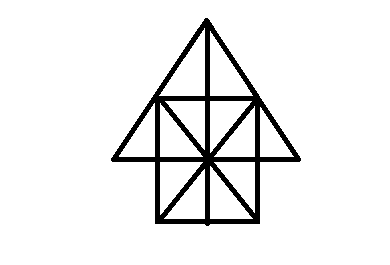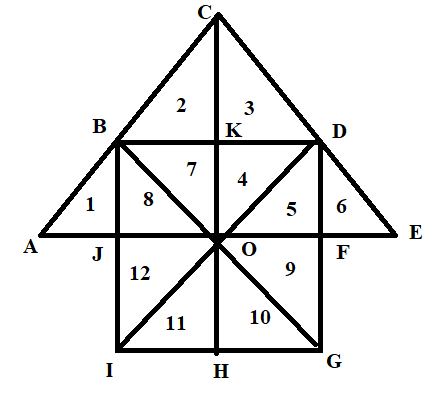• 1
16
Correct
Incorrect
• 2
18
Correct
Incorrect
• 3
25
Correct
Incorrect
• 4
27
Correct
Incorrect
• Workspace
• Discuss

• 1
10
Correct
Incorrect
• 2
8
Correct
Incorrect
• 3
12
Correct
Incorrect
• 4
11
Correct
Incorrect
• Workspace
• Discuss

• 1
4
Correct
Incorrect
• 2
2
Correct
Incorrect
• 3
3
Correct
Incorrect
• 4
5
Correct
Incorrect
• Workspace
• Discuss

• 1
10
Correct
Incorrect
• 2
12
Correct
Incorrect
• 3
14
Correct
Incorrect
• 4
16
Correct
Incorrect
• Workspace
• Discuss

• 1
20
Correct
Incorrect
• 2
24
Correct
Incorrect
• 3
28
Correct
Incorrect
• 4
32
Correct
Incorrect
• Workspace
• Discuss

• 1
16
Correct
Incorrect
• 2
17
Correct
Incorrect
• 3
26
Correct
Incorrect
• 4
30
Correct
Incorrect
• Workspace
• Discuss

• 1
16
Correct
Incorrect
• 2
14
Correct
Incorrect
• 3
8
Correct
Incorrect
• 4
12
Correct
Incorrect
• Workspace
• Discuss

## Q: In question below shown, count the number of triangle and squares.41 05d2c46522bb9b81db4a97ee6

• 1
26 triangles, 5 squares
Correct
Incorrect
• 2
28 triangles, 5 squares
Correct
Incorrect
• 3
26 triangles,6 squares
Correct
Incorrect
• 4
28 triangles, 6 squares
Correct
Incorrect
• Workspace
• Discuss

## Answer : 1. "26 triangles, 5 squares"Explanation :The simplest triangle is shown in figure is 12 and another is IBO, BDO, DGO, GIO, ABO,CBD, DEO, IBD,BDG, DGI, GIB, ACO and COE and ACE is the total no of traingle are 12+14=26Error Reported Successfully

Error Reported Successfully

Error Reported Successfully

Error Reported Successfully

Error Reported Successfully